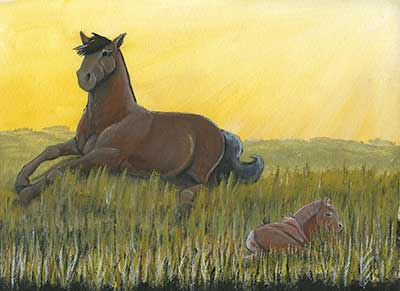# How Do You Measure a Horse?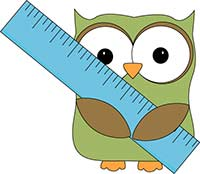## How Do You Measure a Horse?

[cs_undefined row_color=”hsl(82, 39%, 30%)” border_size=”45″ row=”7″]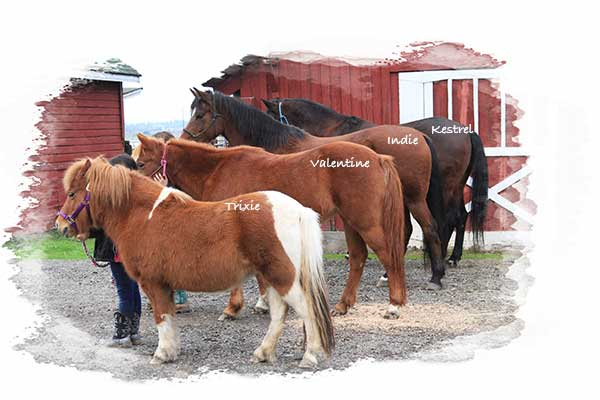Trixie, Valentine, Indie and Kestrel are all different heights:
Trixie is 9.2 Hands
Valentine is 12.2 Hands
Indie is 14.1 Hands
Kestrel is 15 Hands

Why is a horse’s height described in hands? Before there were rulers, people used their hands to measure many different things, including a horse’s height. Four flat fingers or a fist equaled one hand.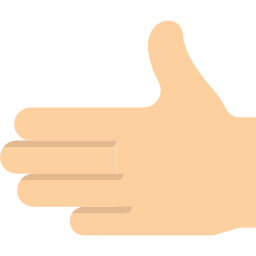Of course, not all hands are the same size, so this type of measurement wasn’t terribly accurate. Two different people could measure the same horse and get different results.

In 1541 in England, King Henry VIII declared that one hand equaled four inches and that is the measurement we use today. So every four inches on a horse is equal to one hand high. For example, Indie is 57 inches tall. If you divide 57 by four you get 14 hands with one inch left over, so we say she is 14.1 hands or 14.1h.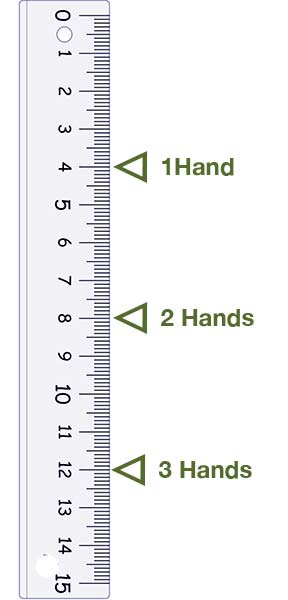Unlike humans, we don’t measure a horse’s height by the top of it’s head. This would be very hard to do because horses move their heads up and down making it difficult to get an accurate measurement. Instead, we measure a horse from the ground to the top of his or her withers. (Learn about horse anatomy here.) In the picture of Kestrel below, the green line shows where to measure a horse.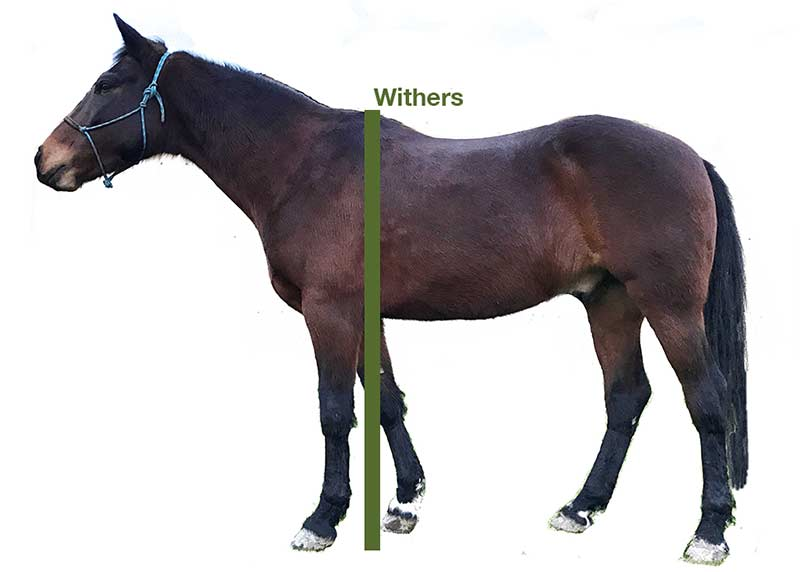## How many hands are you?

To figure out how many hands you are, have someone measure you from head to toe in inches. Divide the total number of inches by four. For example, let’s say you’re 48 inches tall. If you divide that by 4, you get 12, which means you are exactly 12 hands tall. But what if you are 49 inches tall? When you divide 49 by 4, you get 12.25. The .25 is equal to 1 inch, so you are 12.1 hands. If you are 50 inches tall, you are 12.2 hands, and if you are 51 inches tall, you are 12.3 hands tall.

•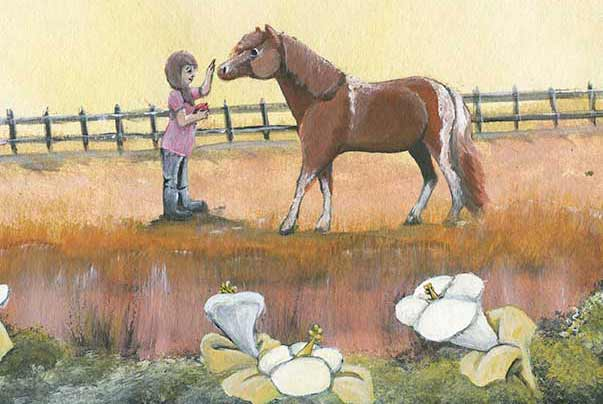•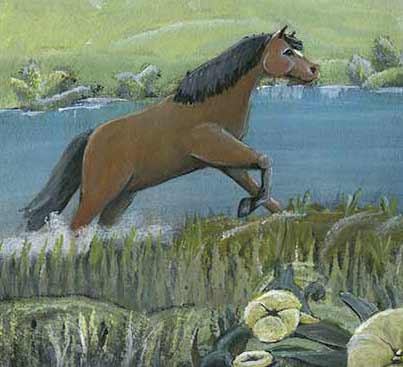•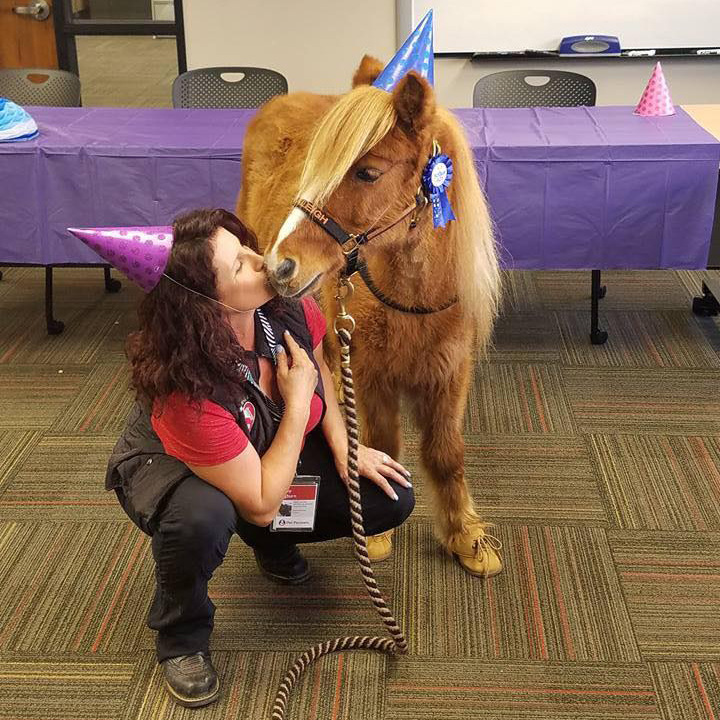••